Bidirectional transfer of quantum information for unknown photons via cross-Kerr nonlinearity and photon-number-resolving measurement
Heo Jino1, 2, Hong Chang-Ho1, 2, Lee Dong-Hoon1, 2, Yang Hyung-Jin1, 3, †,Graduate School of Information Security (GSIS), Korea University, Anam 5-ga Sungbuk-gu, Seoul, Republic of Korea
Center for Information Security Technologies (CIST), Korea University, Seoul, Republic of Korea
Department of Physics, Korea University, Sejong, 339-700, Republic of Korea

† Corresponding author. E-mail: yangh@korea.ac.kr

Abstract
Abstract

We propose an arbitrary controlled-unitary (CU) gate and a bidirectional transfer scheme of quantum information (BTQI) for unknown photons. The proposed CU gate utilizes quantum non-demolition photon-number-resolving measurement based on the weak cross-Kerr nonlinearities (XKNLs) and two quantum bus beams; the proposed CU gate consists of consecutive operations of a controlled-path gate and a gathering-path gate. It is almost deterministic and is feasible with current technology when a strong amplitude of the coherent state and weak XKNLs are employed. Compared with the existing optical multi-qubit or controlled gates, which utilize XKNLs and homodyne detectors, the proposed CU gate can increase experimental realization feasibility and enhance robustness against decoherence. According to the CU gate, we present a BTQI scheme in which the two unknown states of photons between two parties (Alice and Bob) are mutually swapped by transferring only a single photon. Consequently, by using the proposed CU gate, it is possible to experimentally implement the BTQI scheme with a certain probability of success.

1. Introduction

Quantum communication involves the transfer of information (quantum or classical) from one place to another by using quantum phenomena such as quantum key distribution, quantum teleportation,[7,8] quantum secure direct communication, and quantum secret sharing. Quantum operations play a very important role in the experimental realization of optical quantum information processing (QIP) with a certain success probability. Reliable quantum operations can be performed by realizing the photonic multi-qubit gates, such as the arbitrary controlled-unitary (CU) gates.

Cross-Kerr nonlinearities (XKNLs) have been extensively studied both theoretically and experimentally for implementing feasible photonic multi-qubit gates. In principle, because the XKNL effect can induce efficient photon interactions, the photonic multi-qubit gates can be implemented by using far fewer physical resources than linear optical schemes. Nemoto and Munro proposed nearly deterministic controlled gates by using ancilla photons, linear optical elements, and quantum non-demolition (QND) detectors based on weak XKNLs, X-homodyne measurements, and feed-forward schemes. In 2009, Lin and Li presented an almost deterministic controlled-path (C-path) gate by using weak XKNLs and X-homodyne measurements. Thereafter, similar schemes for implementing photonic multi-qubit gates were developed for QIPs via weak XKNLs and X-homodyne measurements. Further, photonic multi-qubit gates, which can reduce the nonlinear phase shift or the strength of coherent state via weak XKNLs, coherent superposition states (CSSs), and P-homodyne measurements for the same error probability, were investigated. These gates not only increase the experimental realization feasibility but also enhance the robustness against decoherence.

However, decoherence (by loss of photons) is unavoidable when a coherent state (probe beam) is transmitted through a fiber in practice. Owing to decoherence, the state of photons (signal) evolves into a mixed state after the homodyne measurements. Consequently, the fidelity of the optical multi-qubit gate will decrease. Fortunately, Jeong has shown that decoherence can be made arbitrarily small simply by increasing the amplitude of the coherent state and by applying the displacement operator D(−α) to the coherent state and photon-number-resolving (PNR) measurements. In 2010, Wittmann et al. showed that the performance of a displacement-controlled PNR measurement is better than that of a homodyne receiver for discriminating the coherent states. Furthermore, the optical multi-qubit or controlled gates, exploiting the QND (PNR) measurement via weak XKNLs and two quantum bus (qubus) beams, were proposed so that XKNL (coupling phase shift) of −θ and the displacement operator D(−α) on the probe beam can be removed.

As mentioned in the above descriptions, the development of multi-qubit gates, in which the XKNLs and various measurement strategies were utilized for deterministic and feasible realization, provides reliable performance and an experimental implementation of optical QIP schemes, such as the quantum communication scheme,[19,23,27] the generation of an entangled state,[22,2426,3941] the deterministic Bell-state measurement,[19,21,42,43] the entanglement purification, and the entanglement concentration. Thereafter, the optical QIP schemes, based on the implementation of multi-qubit gates via weak XKNLs, have been also investigated.

In this paper, first, we propose a deterministic and experimentally feasible CU gate, which is composed of consecutive operations of a C-path gate[34,35] and a gathering-path (G-path) gate via weak XKNLs, the qubus beams, the QND (PNR) measurements, and feed-forward schemes. Compared with the existing multi-qubit gates using XKNLs, coherent states or CSSs and homodyne measurements, for the same error probability, the nonlinear phase shift θ or the amplitude of coherent state α required in our CU gate will be shown to be relatively small. In addition, motivated by Refs. –, we, in our CU gate, utilize the qubus beams and the QND (PNR) measurements without the displacement operator for reducing decoherence (by loss of photons). Thus, when this CU gate is experimentally implemented, the feasibility is improved and decoherence is reduced. Subsequently, by using the proposed CU gates, we present a bidirectional transfer of quantum information (BTQI) for two unknown states of photons that are mutually exchanged between Alice and Bob, by transmitting only one photon.

The rest of this paper is organized as follows. In Section 2, we present a CU gate obtained by consecutive operation of a C-path gate[35,36] and a G-path gate via weak XKNLs, the qubus beams, the QND (PNR) measurements, and feed-forward schemes. Furthermore, we show that when compared with the existing multi-qubit gates using the coherent states or CSSs and homodyne measurements, our CU gate exhibits improved feasibility of the experimental implementation and enhanced robustness against decoherence by using the qubus beams and the QND (PNR) measurements. In Section 3, we propose a BTQI scheme in which two unknown photons between Alice and Bob are mutually transferred by transferring only a single photon via optical elements such as beam splitters (BSs), spin flippers (SFs), polarizing beam splitters (PBSs), 45-PBSs, photon detectors (PDs), and CU gates as described in Section 2. Finally, in Section 4 we discuss the success probability and experimental implementation of the proposed CU gate and BTQI scheme.

2. CU gate with XKNLs, the qubus beams, the QND (PNR) measurements and feed-forwards

Now we consider two types of photon polarizations: circular polarization (|R〉 is right- and |L〉 is left-circular), and linear polarization (|H〉 is horizontal and |V〉 is vertical). The relationships between the two types are given by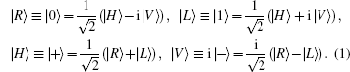Thus, the circularly polarized states correspond to the eigenstates of σZ: {|R〉 ≡ |0〉,|L〉 ≡ |1〉}, and the linearly polarized states correspond to the eigenstates of σX: {|H〉 ≡ |+〉,|V〉 ≡ i |−〉}. We introduce the XKNL for explaining the CU gate. The XKNL’s Hamiltonian is HKerr = ħ χ N1 N2, where Ni is the photon number operator and χ is the strength of nonlinearity in the Kerr medium. We assume that |ni represents a signal state of n photons, and |αj is a coherent state (the probe beam). After passing through the Kerr medium, the signal-probe system state is changed into UKerr |n1 |α2 = ei θ N1 N2 |n1 |α2 = |n1 |α ei 2, where θ = χt and t is the interaction time.

Now, we propose a deterministic CU gate that is composed of consecutively performing C-path gate,[34,35] an arbitrary unitary operator U, and a G-path gate using the XKNLs, the qubus beams, the QND (PNR) measurements, the feed-forwards, and linear optical elements such as 45-PBSs and BSs, which is shown in Fig. 1. Suppose that the initial state of two photons is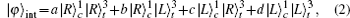where the superscripts describe photon paths and the subscripts c and t represent the control and target photons, respectively.

 Figure Option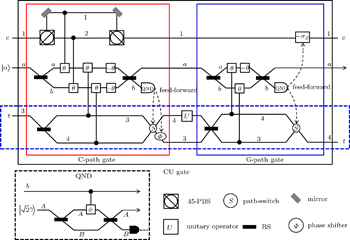Fig. 1. Schematic diagram of CU gate (black box), which is comprised of the consecutive operation of a C-path gate (red box on the left)[34,35] and a G-path gate (blue box on the right) using XKNLs, the qubus beams, the QND (PNR) measurements (dotted line black box), and feed-forwards. The CU gate is composed of three parts: the C-path gate, the operator U, and the G-path gate. First, in the C-path gate, the two photons c and t (dotted line blue box) in the input state (signal) induce controlled phase shifts θ in the two qubus beams (probe) by the XKNLs interaction. Then, the linear phase shifters −θ are operated on the two qubus beams. Subsequently, we measure the probe beam on the path b via the QND (PNR) measurement,[33–38] which can precisely discriminate the number of photons |n〉b in the probe beam on the path b. According to the measurement outcome |n〉b of the QND (PNR) measurement, we determine whether to operate the phase shifter Φ and the path-switch S on the target photon t by feed-forward. After passing the C-path gate, the target photon path is split according to the control photon polarization. The target photon goes through path 3 (or 4) when the control photon is |R〉 (or |L〉). In the second step, the unitary operator U on path 4 is applied to the target photon. In the third step of the G-path gate, the XKNLs induce phase shifts θ according to the target photon t, and then the linear phase shifter −θ is performed on the qubus beam on path a. For gathering the target photon on the split path, we finally apply the unitary operator −σZ to the control photon, and the path-switch S to the target photon via the feed-forward, depending on the measurement outcome |n〉b of the QND (PNR) measurement. Consequently, the CU gate performs a controlled-unitary (CU) operation on the input state.

In the C-path gate[34,35] as shown in Fig. 1, the control photon c passes through the 45-PBS, the target photon t (signal photon) passes through the BS, and the probe beam |α〉 passes through another BS. Then, the state of the signal-probe system is transformed into the following state: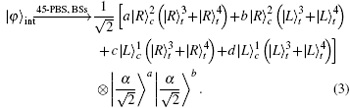When passing through the 45-PBS, |R〉 is transmitted and |L〉 is reflected. The action of 50:50 BS is described by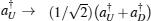and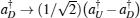, where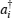is the creation operator of a photon on path i (U is an Up path and D is a Down path). The action of BS transforms the coherent component |αa|βb into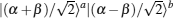. Then, the two photons (c and t) interact with the two qubus beams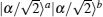to induce the phase shifts θ in Kerr medium.

Subsequently, the linear phase shifters −θ are applied to the qubus beams, and then the signal-probe system is transmitted into 45-PBS (photon c) and BS (two qubus beams). The transformed state |φ01 of the signal-probe system will be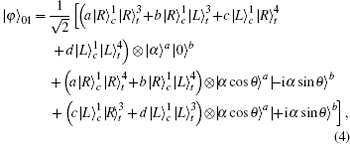where the coherent states on the paths a and b are either |αa|0〉b or |α cos θa |± i α sin θb in the two qubus beams. Then, we employ the QND (PNR) measurement as shown in the dotted line black box in Fig. 1, for precisely measuring the number of photons |nb in the qubus beam (|0〉b or |±α sin θb) on the path b. The measurement is needed for properly operating the path-switch S and the phase shifter Φ by feed-forward. The QND (PNR) measurement operation on the path b is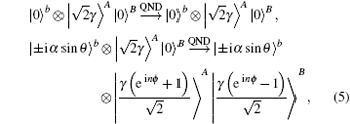where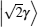is the coherent state and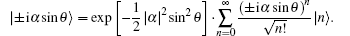By the QND operation, the state |φ01 evolves into the state |φ02 of the signal-probe system and the probe system (path AB), as follows: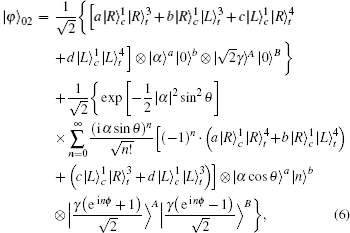where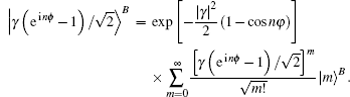We detect the photon number of the state |mB by using the PNR detector which utilizes the positive-operator-value measurement (POVM) elements. The qubus beams on the path B are coupled with the qubus beam |0〉b or |nb in Eq. (6). Such PNR measurement outcomes of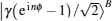in the process of QND can distinguish the Fock state with |nb from that with |n + lb (l is not large enough) in |± iα sinθb. Thus, the QND (PNR) measurement, in which the measurement outcomes |mB ofare detected by POVM elements, will be implemented in the indirect measurement for |nb in the qubus beam on the path b. In Fig. 2, we show an example (PNR measurement using POVM elements) for the Poisson distributions of, which are induced by different |nb (n = 1, 2,…) in the qubus beam on the path b, when |γ| = 100 and ϕ = 0.05.

 Figure Option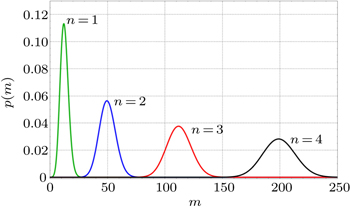Fig. 2. The Poisson distributions of the coupledwith |n〉b (n = 1, 2,…) in the qubus beam on path b, in Eq. (6), by the process of QND, when |γ| = 100 and ϕ = 0.05. m is the photon number and p(m) is the probability to detect the photon number m in the Poisson distribution ofby using the PNR detector. In Eq. (5) with |γ| = 100 and ϕ = 0.05, the overlaps of Poisson distributions for different values of photon numbernare negligible; thus, reliable discrimination between |n〉b (n = 1,2,…) on the path b is possible.

If the QND (PNR) measurement result is |0〉B, the output state |φCP of the C-path gate is the first term in Eq. (6) and is given by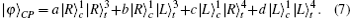Otherwise, the output state can be transformed into the state |φCP, as in Eq. (7), by feed-forward operation of the phase shifter Φ and the path-switch S on the target photon t, according to the |nb (n = 1, 2,…) results of the QND (PNR) measurement. The error probability of the C-path gate,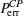, which is the probability to detect |0〉B (no photon) in(n = 0, 1,2,…) on the path B in Eq. (6), is calculated from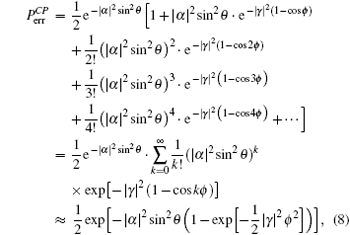where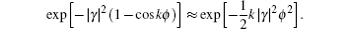When |γ| = 100 and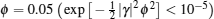in the process of QND (PNR) measurement,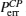is approximated as (1/2)e−|α|2sin2θ. When α sinθα θ > 4.5 (α ∈ ℝ),is smaller than 10− 9; thus, the deterministic C-path gate is possible. It indicates that if we choose the amplitude α of the coherent state to be sufficiently large, the weak XKNL (θ ≪ 1) can be utilized for the C-path gate. Thus, by using weak XKNLs, the qubus beams, and the QND (PNR) measurement with the condition α θ > 4.5, this C-path gate is nearly deterministic with a certain success probability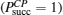. After passing the C-path gate, the signal-probe system |ψCP, in which the target photon’s path is split as shown in Eq. (7), including the unmeasured qubus beam |αa or |α cosθa on the path a, is given by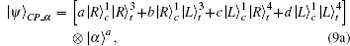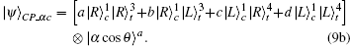If the QND measurement outcome of the coherent state on the path B in the C-path gate is |0〉B, the qubus beam on the path a of the state |ψCP is |αa, as in Eq. (9a). If the measurement outcome is |nB (n = 1,2,…), the qubus beam is |α cosθa, as in Eq. (9b). Subsequently, the qubus beams |αa or |α cosθa in the state |ψCP will be recycled in the G-path gate.

In the second step, the target photon in the state |ψCP, in Eq. (9a) or (9b), passes through an arbitrary unitary operator U on path 4. The transformed state |ψU is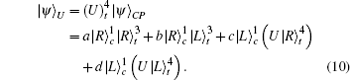For example, if U is σX, σZ or iσY, the state |ψU will be given by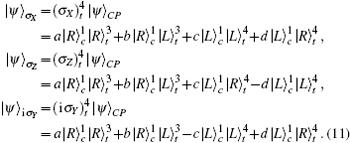These are the output states of the unitary operation of CNOT, controlled-σZ (CZ) or controlled-σY (CY) on the initial state of the two photons (Eq. (2)), respectively. Since the target photon path is divided into two paths 3 and 4 in Eq. (11), we use the G-path gate for merging the target photon split path to a single path.

In the G-path gate of the third step as described in Fig. 1, the qubus beams |αa or |α cosθa, which were used in the C-path gate, pass through the BS, and the target photon t in the state |ψU passes through another BS. After passing the BS, the qubus beams |αa or |α cosθa are split into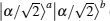or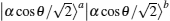, and their phase is shifted through the XKNLs interaction in which the target photon t induces a controlled phase shift θ on a qubus beam. Subsequently, the linear phase shifter −θ is applied to the qubus beam on the path a, and the two qubus beams are transmitted to the BS. The transformed state |ψ01 of the signal-probe system will be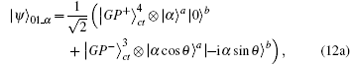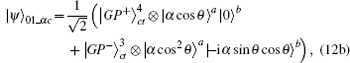where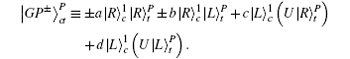Then, we employ the QND (PNR) measurement, as shown in the dotted line black box in Fig. 1, for precisely measuring the outcomes of the qubus beams on the path b. The measurement is needed for properly operating the path-switch S and Pauli operator −σZ by feed-forward. The QND (PNR) measurement operation is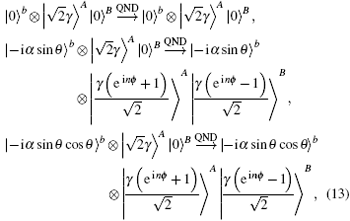where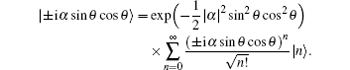After the operation of QND, the output state |ψ02 is given by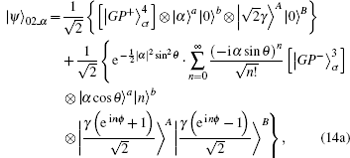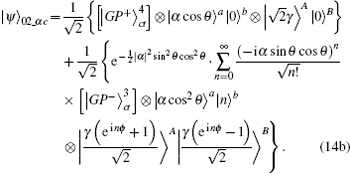Subsequently, we detect the photon number of the state |mB by using the PNR detector using POVM elements. The qubus beam states on the path B are coupled with the qubus beams |0〉b or |nb in Eq. (14a) or (14b). Such PNR measurement outcomes ofin the process of QND can distinguish the Fock state with |nb from that with |n + lb (l is not large enough) in |− iα sinθb or |− iα sinθ cosθb. If the result of QND (PNR) measurement is |0〉B, the final state |ψGP of the G-path gate is the first term in Eq. (14a) or (14b) and is given by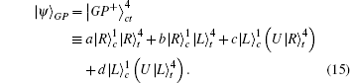Otherwise, the output state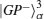can be transformed into the final state |ψGP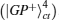, as in Eq. (15), by operating the path-switch S on the target photon t, and −σZ on the control photon c via the feed-forward scheme, according to the |nb (n = 1, 2,…) results of the QND (PNR) measurement. After passing the G-path gate, the error probability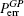of this gate according to the state |ψ02_α (|ψ02_αc) is (1/2)e−|α|2sin2θ[(1/2)e−|α|2sin2θ cos2θ]. When the weak XKNL (θ ≪ 1) is employed in this gate, (1/2)e−|α|2sin2θ cos2θ is almost identical to (1/2)e−|α|2sin2θ; thus, the error probabilityis also the same as the error probability of the C-path gate; thus,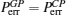. Consequently, the total error probability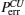of the single CU gate, which is composed of consecutive operations of C- and G-path gates, is given by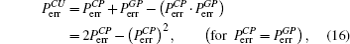where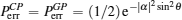for the weak XKNL (θ ≪ 1), and the strong amplitude of coherent state (α ≫ 1). Thus, the CU gate is nearly deterministic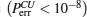for α sinθα θ > 4.5. Furthermore, our multi-qubit gate, using two qubus beams and the QND (PNR) measurement, is more experimentally efficient than both the existing multi-qubit gates using coherent states and X-homodynes, or those using CSS and P-homodynes. Compared with our C-path gate, the existing C-path gates using coherent states and X-homodynes, or using CSS and P-homodynes, have the following error probabilities:where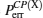and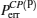are the error probabilities of the C-path gates using coherent state and X-homodyne and using CSS and P-homodyne, respectively.

Table 1 presents the comparison between our proposed C-path gate and the existing C-path gates.[23,27] If we choose the same error probability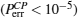, the proposed method using two qubus beams and QND (PNR) measurements can reduce the nonlinear phase shift (if α = 1000, then θ > 0.0033) or the strength of the coherent state (if θ ≈ 10− 2, then α > 370). Thus, compared with the existing C-path gates[23,27] employing the measurement strategy involving homodynes (X and P), the proposed CU gate increases the feasibility of experimental realization in terms of the resources consumed, owing to the PNR measurement. Moreover, the decoherence in the C-path gate reported in Ref. , which uses the probe beam by CSS (macroscopic system), is far larger than that in our proposed gate employing the coherent state. Consequently, the proposed CU gate not only increases the feasibility of experimental realization but also enhances the robustness against decoherence.Table 1.

Comparison of the C-path gates proposed in this paper with the gates reported in Refs.  and . We compare the used probe beam, measurement strategy, and error probability of the gates. The requirements (α,θ) are calculated for the same error probability: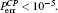.
3. Bidirectional transfer of quantum information (BTQI) via CU gates using XKNLs, the qubus beams, and the QND (PNR) measurements

The BTQI scheme as described in Fig. 3, achieves the mutual transfer of two unknown states of photon between Alice and Bob by transferring only a single photon T. The unknown states are prepared as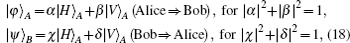where the polarizations {|H〉,|V〉}, {|R〉,|L〉} are defined by Eq. (1). suppose that Alice prepares a photon A in an unknown state |φA and a photon T in the state |HT. After the photon T (on the path P) passes through the BS, Alice operates optical devices SF, WPs, CNOT, and CZ gates between the photons T and A. The output state |ΦAlice of the photon system (TA) is obtained as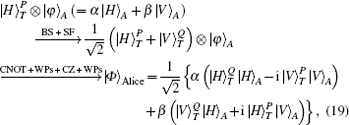where the photon T acts as a control photon and the photon A is used as a target photon in CNOT and CZ gates, as in Eq. (11). The operations UWP of WP and USF of SF are described in Fig. 3.

 Figure Option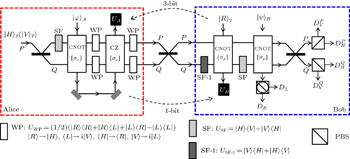Fig. 3. The BTQI scheme, which consists of a BS, an SF, wave-plates (WPs), a CNOT gate, and a CZ gate on Alice’s side. On Bob’s side, it has an SF-1, an SF, a BS, PBSs, a 45-PBS, PDs (D), and two CNOT gates. The state |φ〉A (|ψ〉B) is an unknown state of the photon that Alice (Bob) wants to send to Bob (Alice). The state|φ〉A (|ψ〉B) is recovered by Bob (Alice) by using proper unitary operation UB (UA). The suitable operation is determined by the information about the initial polarization of the transferred photon T (1 bit), the measured path and polarization of the transferred photon T, and the measured polarization of photon B in PDs (3 bits). Subsequently, the state of Alice’s photon A is reconstructed at the final destination of Bob’s photon 2, (|φ〉A → |R〉2), and the state of Bob’s photon B is reconstructed at the final destination of Alice’s photon A, (|ψ〉B → |φ〉A).

In Fig. 4, the CU operations which Alice and Bob use for transferring unknown photons in the BTQI scheme are performed by the CU gates consisting of a C-path gate and a G-path gate via XKNLs, the qubus beams, the QND (PNR) measurements, and feed-forwards, as described in Section 2. Subsequently, Alice transmits the photon T of the state |ΦAlice to Bob, while the photon A remains on Alice’s side.

 Figure Option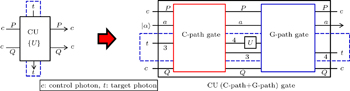Fig. 4. Photon T is used as a control qubit, and the photons A, 2, and B are used as target qubits in the CU operations. These CU operations are implemented by the CU gates, in which the C-path and G-path gates are consecutively performed by XKNLs, the qubus beams, the QND (PNR) measurements, and feed-forwards, as described in Section 2. If the unitary operator U in the middle between the C-path and G-path gates is σX the CU gate will become a CNOT, whereas if the operator is σZ the CU gate will become a CZ. For the BTQI scheme, the details of this CU gate are presented in Appendix A.

On Bob’s side, after the photon T of the state |ΦAlice passes through the BS, Bob performs the SF-1 (USF − 1) as described in Fig. 3, and the first CNOT gate between the photon T (control) and |R2 (target) for reconstructing Alice’s unknown state |φA. This resulting state |ΦTA2 of photons is achieved as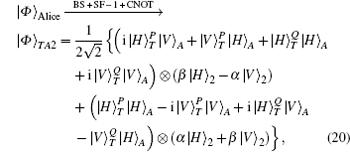where the expansion coefficients α and β of Alice’s unknown photon A appear in Bob’s photon state 2. After this state |ΦTA2 passes through the SF, the second CNOT gate between the photon T (control) and B (target) is applied for transferring Bob’s unknown state |ψB (= χ |HB + δ |VB) to Alice. Then, the photon T passes through the BS. Consequently, when Alice prepares the initial state of photon |HT in Eq. (19), the final state |ΦBob_H of the photon system (TAB ⊗2) is given by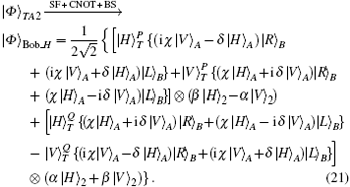If Alice transfers the polarization |VT of the prepared photon to Bob along path P,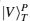, and if Alice (Bob) wants to send |φA (|ψB), as described in Eq. (18), to Bob (Alice), the final state |ΦBob_V would be expressed as follows: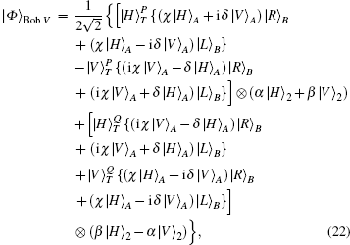where the photon B in Eqs. (21) and (22) is circularly polarized {|R〉,|L〉}.

To measure the paths and polarizations of the photons, Bob transmits the photon T to the PBSs and the photon B to the 45-PBS. Because |H〉 (|R〉) is transmitted and |V〉 (|L〉) is reflected by the PBS (45-PBS), Bob can measure both the path and the polarization of photon T in the {|HT,|VT} basis by using PDs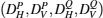and the polarization of photon B in the {|RB,|LB} basis by using PDs (DR,DL). However, Bob does not measure the polarization of photon 2. After these measurements, the photon state 2 is collapsed into one of two possible states and the photon state A is also collapsed into one of eight possible states. Subsequently, Alice announces to Bob the initial polarization information (1 bit) of the transferred photon T, and Bob communicates to Alice the measured path and polarization information (3 bits) of photons T and B, via the public channel. By performing proper operations UA on Alice’s target photon A and by performing UB on Bob’s target photon 2, both can recover the other’s unknown states of photons, χ |HA + δ |VA and α |H2 + β |V2. In Table 2 summarized are all possible states of Alice and Bob’s target photons (photons A and 2) and the suitable unitary operators (UA and UB), which depend on the initial polarization information of the transferred photon T and the measured path and polarizations of photons T and B. Furthermore, the security against Eve’s intercept-resend attack of the BQTI scheme is guaranteed if Alice (Bob) uses a secure classical channel (instead of public channel) to notify the initial polarization of the transferred photon T (the measured path and polarization of photons T and B). This is because Eve cannot reconstruct the quantum states of unknown photons without knowing information (1, 3 bits) about the path and polarizations of photons T and B. When Eve, impersonating Bob, intercepts the photon T being transferred from Alice to Bob and measures the path of this photon and the polarization of photon B, like Bob, photon 2 is collapsed into one of four possible states, as shown in Table 2. She cannot determine which of these collapsed states is without the information about the initial polarization. If Eve impersonates Alice, Eve cannot recover Bob’s unknown state of photon B from the information about photon A without Bob’s measurement outcomes. Thus, Alice and Bob can achieve the secure quantum transmission of unknown photons with the secure classical channel. Accordingly, the secure BTQI scheme involves the bidirectional quantum transmission of unknown photons by transferring only a single photon T and using CU gates as described in Section 2.Table 2.

Collapsed states of the photons (photons A and 2) and the suitable unitary operations (UA,UB), depending on Alice’s initial polarization and Bob’s measurement outcomes.

.
4. Conclusions

In this paper, we propose a BTQI scheme, which achieves mutual transmission of two unknown states |φA,|ψB of photons between Alice and Bob, which do not directly send unknown states of photons (A,B) but instead use a classical channel to send the information about the states of photons and then utilize the information to reconstruct the unknown states. In our protocol, both Alice’s unknown state |φA and Bob’s unknown state |ψB are simultaneously transmitted to the other’s site. Thus, the two participants in our protocol simultaneously become both message senders and message receivers by directly transferring only a single photon T.

Because the BTQI scheme is based on the CU gate, the success probability and feasibility of realization of the proposed scheme (BTQI) strongly depend on the nearly deterministic performance and the experimental implementation of the CU gate, which can be operated by the consecutive performances of C-path and G-path gates via weak XKNLs, the qubus beams, the QND (PNR) measurements, and feed-forwards, as described in Section 2. The total error probability of the single CU gate is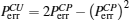, where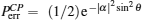as given by Eq. (16). For α sinθα θ > 4.5, the CU gate has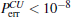and thus is nearly deterministic. Further, the XKNL is not necessarily very large (θ ≪ 1) because it can be compensated for by using the coherent state |α〉 with very large amplitude. From this point of view (a weak XKNL and a large amplitude of coherent state), our CU gate uses weaker XKNL or smaller amplitude of the probe beam than the existing multi-qubit gates based on the coherent state and X-homodyne or CSS and P-homodyne.

In Fig. 5, for the same error probabilities as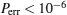, if the amplitude of the probe beam in the multi-qubit gate is α = 1000, the amplitude of XKNLs in our C-path gate is θ > 0.0033, smaller than that of the existing C-path gate based on the coherent state and X-homodyne (θ > 0.094) or CSS and P-homodyne (θ > 0.0045). Although the natural XKNLs are extremely weak, θ ≈ 10− 18, it has been suggested that the amplitude of the nonlinearity could be significantly improved by using electromagnetically induced transparency (EIT), say θ ≈ 10−2,[53,54] which is effectively employed in the multi-qubit gates. When the nonlinear phase shift θ is 10− 2, the amplitudes of probe beams in our CU gate should be α > 360, as indicated in Eq. (8), in the existing multi-qubit gates using X-homodyne it should be α >95000, and in the gates using P-homodyne it should be α > 480, as indicated in Eq. (17), for the same error probabilities as Perror < 10− 6.

 Figure Option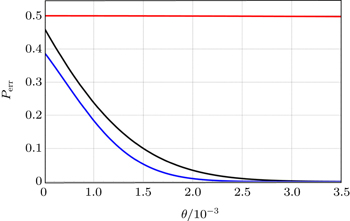Fig. 5. Plots of the error probabilities of the C-path gates based on coherent state and X-homodyne (red line(upper)), CSS and P-homodyne (black curve (middle)), and the qubus beams and the QND (PNR) detection (blue curve (lower)), each as a function of θ (the XKNL’s phase shift), for probe beam amplitude α = 1000. The red (upper), black (middle), and blue (lower) plots are the functions of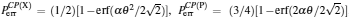and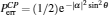respectively, as indicated in Eq. (19). For example, when θ = 0.003 and α = 1000, the error probabilities are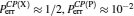, and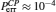.

In experimental implementation of multi-qubit gates, the fidelity of multi-qubit gates with using the homodyne measurement strategy will be reduced, owing to the photon loss-related decoherence. However, the proposed CU gate can reduce the decoherence by increasing the coherent state amplitude and using the PNR measurements. Furthermore, our CU gate based on the qubus beams does not need to perform the displacement operator D(− α), and XKNL (coupling phase shift) of −θ. The minus conditional phase shift in XKNL is known to be difficult to implement practically. Another advantage of the devised CU gate, which is composed of consecutive operations of C-path and G-path, is that the qubus beam |αa that is employed in the C-path gate can be recycled (|αa or |α cosθa) in the G-path gate. In practice, the strength of the XKNL can be amplified by using EIT,[53,54] whispering-gallery mode micro-resonators,[56,57] optical fibers, and cavity QED.[59,60] These methods can also reduce the absorption or losses of signal photons and the probe beam (coherent state) under a weak XKNL (θ ≪ 1). Moreover, references  and  show the feasibility of the large nonlocal nonlinear interactions in a Rydberg EIT system. For generating a large XKNL between co-propagating weak pulses in 87Rb, Wang et al. proposed the use of double EIT. Thus, the sufficient strength of the XKNL for optical QIP is expected in the implementation of the realization.

Consequently, the proposed CU gate, in which used are the weak XKNLs, two qubus beams, QND (PNR) measurements and feed-forwards, is almost deterministic with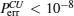for α θ > 4.5, and can reduce the nonlinear phase shift θ or the strength of the probe beam α compared with the existing multi-qubit gates based on the coherent state and X-homodyne or those based on the CSSs and P-homodyne. In addition, by employing the strategy of PNR measurements, our CU gate can reduce the decoherence effect, and by employing the qubus beams we can recycle the qubus beam in the G-path gate. Thus, the designed CU gate can improve the feasibility and efficiency of experimental realization and augment the robustness against decoherence by using the proposed techniques. In conclusion, our BTQI scheme is experimentally applicable with a certain success probability.

Reference
 1 Ekert A K 1991 Phys. Rev. Lett. 67 661 2 Long G LLiu X S 2002 Phys. Rev. A 65 032302 3 Hong C HHeo JKhym G LLim J IHong S KYang H J 2010 Opt. Commun. 283 2644 4 Zhang C MLi MHuang J ZTreeviriyanupab PLi H WLi F YWang CYin Z QChen WSripimanwat KHan Z F 2014 Chin. Sci. Bull. 59 2825 5 Zhao L YLi H WYin Z QChen WYou JHan Z F 2014 Chin. Phys. B 23 100304 6 Li F YYin Z QLi H WChen WWang SWen HZhao Y BHan Z F 2014 Chin. Phys. Lett. 31 070302 7 Bennett C HBrassard GCrepeau CJozsa RPeres AWootters W K 1993 Phys. Rev. Lett. 70 1895 8 Heo JHong C HLim J IYang H J 2013 Chin. Phys. Lett. 30 040301 9 Bostrom KFelbinger F 2002 Phys. Rev. Lett. 89 187902 10 Deng F GLong G LLiu X S 2003 Phys. Rev. A 68 042317 11 Hong C HHeo JLim J IYang H J 2014 Chin Phys. B 23 090309 12 Zheng CLong G F 2014 Sci. China-Phys. Mech. Astron. 57 1238 13 Hong C HHeo JLim J IYang H J2010Chin. Phys. Lett.29050303 14 Knill ELaflamme RMilburn G J 2001 Nature 409 46 15 Zhao ZZhang A NChen Y AZhang HDu J FYang TPan J W 2005 Phys. Rev. Lett. 94 030501 16 Langford N KWeinhold T JPrevedel RResch K JGilchrist AO’Brien J LPryde G JWhite A G 2005 Phys. Rev. Lett. 95 210504 17 Nemoto KMunro W J 2004 Phys. Rev. Lett. 93 250502 18 Lin QLi J 2009 Phys. Rev. A 79 022301 19 Guo QBai JCheng L YShao X QWang H FZhang S 2011 Phys. Rev. A 83 054303 20 Zhao R TGuo QCheng L YSun L LWang H FZhang S 2013 Chin. Phys. B 22 030313 21 Barrett S DKok PNemoto KBeausoleil R GMunro W JSpiller T P 2005 Phys. Rev. A 71 060302 22 Kang Y HXia YLu P M 2014 Int. J. Theor. Phys. 53 17 23 Heo JHong C HLim J IYang H J 2015 Chin. Phys. B 24 050304 24 Jin G SLin YWu B 2007 Phys. Rev. A 75 054302 25 Zheng C HZhao JShi PLi W DGu Y J 2014 Opt. Commun. 316 26 26 Yan XYu Y FZhang Z M 2014 Chin. Phys. B 23 060306 27 Heo JHong C HLim J IYang H J 2015 Int. J. Theor. Phys. 54 2261 28 Munro W JNemoto KSpiller T P 2005 New J. Phys. 7 137 29 Jeong H 2005 Phys. Rev. A 72 034305 30 Jeong H 2006 Phys. Rev. A 73 052320 31 Barrett S DMilburn G J 2006 Phys. Rev. A 74 060302 32 Wittmann CAndersen U LTakeoka MSych DLeuchs G 2010 Phys. Rev. A 81 062338 33 He BRen YBergou J A 2009 Phys. Rev. A 79 052323 34 Lin QHe B 2009 Phys. Rev. A 80 042310 35 Lin QHe BBergou J ARen Y 2009 Phys. Rev. A 80 042311 36 Su S LZhu LGuo QWang H FZhu A DZhang SYeon K H 2012 Opt. Commun. 285 4134 37 Zhu M ZYe L 2014 J. Opt. Soc. Am. B 31 405 38 Zhu M ZYe L 2015 Opt. Commun. 334 51 39 Dong LXiu X MGao Y JYi X X 2013 Quantum. Inform. Process. 12 1787 40 Yan XYu Y FZhang Z M 2014 Chin. Phys. B 23 060306 41 Han XHu SGuo QWang H FZhang S 2015 Quantum. Inform. Process. 14 1919 42 Zhou JYang MLu YCao Z L 2009 Chin. Phys. Lett. 26 100301 43 Sheng Y BDeng F GLong G L 2010 Phys. Rev. A 82 032318 44 Sheng Y BDeng F GZhou H Y 2008 Phys. Rev. A 77 042308 45 Sheng Y BZhou L2015Sci. Rep.1513453 46 Sheng Y BDeng F G 2010 Phys. Rev. A 81 032307 47 Liu H JFan L LXia YSong J 2015 Quantum. Inform. Process. 14 2909 48 Sheng Y BZhou LZhao S M 2012 Phys. Rev. A 85 042302 49 Sheng Y BZhou L2015Sci. Rep.157815 50 Loudon R2000The Quantum Theory of LightOxfordOxford university press 51 Kok PBraunstein S L 2000 Phys. Rev. A 61 042304 52 Kok PMunro W JNemoto KRalph T CDowing J PMilburn G J 2007 Rev. Mod. Phys. 79 135 53 Lukin M DLu A I 2000 Phys. Rev. Lett. 84 1419 54 Lukin M DLu A I 2001 Nature 413 273 55 Kok P 2008 Phys. Rev. A 77 013808 56 Kippenberg TSpillane SVahala K 2004 Phys. Rev. Lett. 93 083904 57 Wang X WTang S QZhang D Y 2013 Opt. Commun. 296 153 58 Li XVoss PSharping JKumar P 2005 Phys. Rev. Lett. 94 053601 59 Grangier PLevenson J APoizat J P 1998 Nature 396 537 60 Hofmann H FKojima KTakeuchi SSasaki K 2003 J. Opt. B 5 218 61 He BMacRae AHan YLvovsky ASimon C 2011 Phys. Rev. A 83 022312 62 Shahmoon EKurizki GFleischhauer MPetrosyn 2011 Phys. Rev. A 83 033806 63 Wang Z BMarzlin K PSanders B C 2006 Phys. Rev. Lett. 97 063901